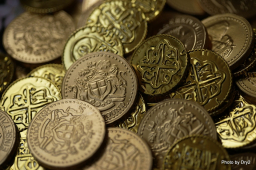# Assume

Assume that you are to buy 5-peso worth of candy on two different stores. In your coin purse that contains two 20-peso coin, three 10-peso coin, six 5-peso coin, and seven 1-peso coin, what is the probability of getting two consecutive 5-peso coin from your purse assuming that you already paid the first store a 5-peso coin before getting another 5-peso coin from your purse to pay the second store?

p =  0.098

### Step-by-step explanation:Did you find an error or inaccuracy? Feel free to write us. Thank you!

Tips for related online calculators
Need help calculating sum, simplifying, or multiplying fractions? Try our fraction calculator.
Would you like to compute the count of combinations?